<![CDATA[Journal of the Australian Mathematical Society]]>https://www.cambridge.org/core/journals/journal-of-the-australian-mathematical-society/latest-issueJournal of the Australian Mathematical Society1446788714468107Cambridge University Press<![CDATA[A RESULT OF PALEY AND WIENER ON DAMEK–RICCI SPACES]]>

A classical result due to Paley and Wiener characterizes the existence of a nonzero function in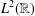, supported on a half-line, in terms of the decay of its Fourier transform. In this paper, we prove an analogue of this result for Damek–Ricci spaces.

]]>
https://dx.doi.org/10.1017/S1446788719000077?rft_dat=source%3Ddrssdoi:10.1017/S1446788719000077A RESULT OF PALEY AND WIENER ON DAMEK–RICCI SPACES11610.1017/S1446788719000077https://dx.doi.org/10.1017/S1446788719000077?rft_dat=source%3Ddrss2019-05-03Journal of the Australian Mathematical Society2019-05-03BHOWMIK, MITHUN
<![CDATA[GROUP ALGEBRAS WHOSE UNIT GROUP IS LOCALLY NILPOTENT]]>

We present a complete list of groups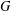and fields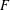for which: (i) the group of normalized units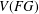of the group algebra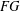is locally nilpotent; (ii) the set of nontrivial nilpotent elements ofis finite and nonempty, and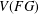is an Engel group.

]]>
https://dx.doi.org/10.1017/S1446788719000557?rft_dat=source%3Ddrssdoi:10.1017/S1446788719000557GROUP ALGEBRAS WHOSE UNIT GROUP IS LOCALLY NILPOTENT172310.1017/S1446788719000557https://dx.doi.org/10.1017/S1446788719000557?rft_dat=source%3Ddrss2020-05-07Journal of the Australian Mathematical Society2020-05-07BOVDI, V.
<![CDATA[ON THE NUMBER OF SUBSEMIGROUPS OF DIRECT PRODUCTS INVOLVING THE FREE MONOGENIC SEMIGROUP]]>

The direct product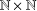of two free monogenic semigroups contains uncountably many pairwise nonisomorphic subdirect products. Furthermore, the following hold for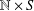, where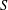is a finite semigroup. It contains only countably many pairwise nonisomorphic subsemigroups if and only ifis a union of groups. And it contains only countably many pairwise nonisomorphic subdirect products if and only if every element ofhas a relative left or right identity element.

]]>
https://dx.doi.org/10.1017/S1446788718000605?rft_dat=source%3Ddrssdoi:10.1017/S1446788718000605ON THE NUMBER OF SUBSEMIGROUPS OF DIRECT PRODUCTS INVOLVING THE FREE MONOGENIC SEMIGROUP243510.1017/S1446788718000605https://dx.doi.org/10.1017/S1446788718000605?rft_dat=source%3Ddrss2019-02-01Journal of the Australian Mathematical Society2019-02-01CLAYTON, ASHLEYRUŠKUC, NIK
<![CDATA[GENERALIZED JORDAN DERIVATIONS ON SEMIPRIME RINGS]]>

The purpose of this note is to prove the following. Suppose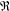is a semiprime unity ring having an idempotent element(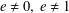) which satisfies mild conditions. It is shown that every additive generalized Jordan derivation onis a generalized derivation.

]]>
https://dx.doi.org/10.1017/S1446788719000259?rft_dat=source%3Ddrssdoi:10.1017/S1446788719000259GENERALIZED JORDAN DERIVATIONS ON SEMIPRIME RINGS364310.1017/S1446788719000259https://dx.doi.org/10.1017/S1446788719000259?rft_dat=source%3Ddrss2019-07-29Journal of the Australian Mathematical Society2019-07-29FERREIRA, BRUNO L. M.FERREIRA, RUTH N.GUZZO, HENRIQUE
<![CDATA[STOCHASTIC NONLINEAR SCHRÖDINGER EQUATION WITH ALMOST SPACE–TIME WHITE NOISE]]>

We study the stochastic cubic nonlinear Schrödinger equation (SNLS) with an additive noise on the one-dimensional torus. In particular, we prove local well-posedness of the (renormalized) SNLS when the noise is almost space–time white noise. We also discuss a notion of criticality in this stochastic context, comparing the situation with the stochastic cubic heat equation (also known as the stochastic quantization equation).

]]>
https://dx.doi.org/10.1017/S1446788719000156?rft_dat=source%3Ddrssdoi:10.1017/S1446788719000156STOCHASTIC NONLINEAR SCHRÖDINGER EQUATION WITH ALMOST SPACE–TIME WHITE NOISE446710.1017/S1446788719000156https://dx.doi.org/10.1017/S1446788719000156?rft_dat=source%3Ddrss2019-06-21Journal of the Australian Mathematical Society2019-06-21FORLANO, JUSTINOH, TADAHIROWANG, YUZHAO
<![CDATA[PERFECT TRIANGLES ON THE CURVE $C_{4}$ ]]>

A Heron triangle is a triangle that has three rational sides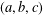and a rational area, whereas a perfect triangle is a Heron triangle that has three rational medians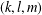. Finding a perfect triangle was stated as an open problem by Richard Guy [Unsolved Problems in Number Theory (Springer, New York, 1981)]. Heron triangles with two rational medians are parametrized by the eight curves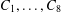mentioned in Buchholz and Rathbun [‘An infinite set of heron triangles with two rational medians’, Amer. Math. Monthly 104(2) (1997), 106–115; ‘Heron triangles and elliptic curves’, Bull. Aust. Math.Soc. 58 (1998), 411–421] and Bácskái et al. [Symmetries of triangles with two rational medians, http://citeseerx.ist.psu.edu/viewdoc/summary?doi=10.1.1.65.6533, 2003]. In this paper, we reveal results on the curve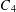which has the property of satisfying conditions such that six of seven parameters given by three sides, two medians and area are rational. Our aim is to perform an extensive search to prove the nonexistence of a perfect triangle arising from this curve.

]]>
https://dx.doi.org/10.1017/S144678871900003X?rft_dat=source%3Ddrssdoi:10.1017/S144678871900003XPERFECT TRIANGLES ON THE CURVE $C_{4}$ 688010.1017/S144678871900003Xhttps://dx.doi.org/10.1017/S144678871900003X?rft_dat=source%3Ddrss2019-10-09Journal of the Australian Mathematical Society2019-10-09ISMAIL, SHAHRINA
<![CDATA[COMPLETE WEINGARTEN HYPERSURFACES SATISFYING AN OKUMURA TYPE INEQUALITY]]>

In this paper we deal with complete linear Weingarten hypersurfaces immersed into Riemannian space forms. Assuming an Okumura type inequality on the total umbilicity tensor of such hypersurfaces, we prove that either the hypersurface is totally umbilical or it holds an estimate for the norm of the total umbilicity tensor, which is also shown be sharp in the sense that the product of space forms realize them. Our approach is based on a version of the Omori–Yau maximum principle for a suitable Cheng–Yau type operator.

]]>
https://dx.doi.org/10.1017/S1446788719000028?rft_dat=source%3Ddrssdoi:10.1017/S1446788719000028COMPLETE WEINGARTEN HYPERSURFACES SATISFYING AN OKUMURA TYPE INEQUALITY819210.1017/S1446788719000028https://dx.doi.org/10.1017/S1446788719000028?rft_dat=source%3Ddrss2019-03-11Journal of the Australian Mathematical Society2019-03-11DE LIMA, EUDES L.DE LIMA, HENRIQUE F.
<![CDATA[ALGEBRAIC CUNTZ–KRIEGER ALGEBRAS]]>

We show that a directed graph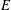is a finite graph with no sinks if and only if, for each commutative unital ring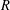, the Leavitt path algebra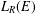is isomorphic to an algebraic Cuntz–Krieger algebra if and only if the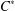-algebra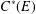is unital and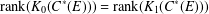. Letbe a field and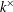be the group of units of. When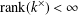, we show that the Leavitt path algebra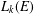is isomorphic to an algebraic Cuntz–Krieger algebra if and only if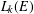is unital and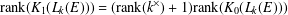. We also show that any unital-algebra which is Morita equivalent or stably isomorphic to an algebraic Cuntz–Krieger algebra, is isomorphic to an algebraic Cuntz–Krieger algebra. As a consequence, corners of algebraic Cuntz–Krieger algebras are algebraic Cuntz–Krieger algebras.

]]>
<![CDATA[HYPERREFLEXIVITY CONSTANTS OF THE BOUNDED $N$ -COCYCLE SPACES OF GROUP ALGEBRAS AND C*-ALGEBRAS]]>

We introduce the concept of strong property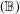with a constant for Banach algebras and, by applying a certain analysis on the Fourier algebra of the unit circle, we show that all C*-algebras and group algebras have the strong propertywith a constant given by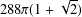. We then use this result to find a concrete upper bound for the hyperreflexivity constant of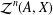, the space of bounded-cocycles from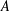into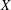, whereis a C*-algebra or the group algebra of a group with an open subgroup of polynomial growth andis a Banach-bimodule for which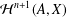is a Banach space. As another application, we show that for a locally compact amenable groupand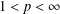, the space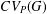of convolution operators on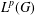is hyperreflexive with a constant given by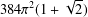. This is the generalization of a well-known result of Christensen [‘Extensions of derivations. II’, Math. Scand. 50(1) (1982), 111–122] for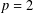.

]]>
https://dx.doi.org/10.1017/S1446788719000089?rft_dat=source%3Ddrssdoi:10.1017/S1446788719000089HYPERREFLEXIVITY CONSTANTS OF THE BOUNDED $N$ -COCYCLE SPACES OF GROUP ALGEBRAS AND C*-ALGEBRAS11213010.1017/S1446788719000089https://dx.doi.org/10.1017/S1446788719000089?rft_dat=source%3Ddrss2019-04-30Journal of the Australian Mathematical Society2019-04-30SAMEI, EBRAHIMFARSANI, JAFAR SOLTANI
<![CDATA[A MODULAR PROOF OF TWO OF RAMANUJAN’S FORMULAE FOR $1/\unicode[STIX]{x1D70B}$ ]]>

In this article, we give new proofs of two of Ramanujan’s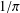formulae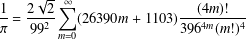and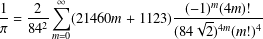using the theory of modular forms. The method can also be used to prove other classicalformulae.

]]>
https://dx.doi.org/10.1017/S1446788718000599?rft_dat=source%3Ddrssdoi:10.1017/S1446788718000599A MODULAR PROOF OF TWO OF RAMANUJAN’S FORMULAE FOR $1/\unicode[STIX]{x1D70B}$ 13114410.1017/S1446788718000599https://dx.doi.org/10.1017/S1446788718000599?rft_dat=source%3Ddrss2019-02-01Journal of the Australian Mathematical Society2019-02-01ZHAO, YUE
<![CDATA[JAZ volume 109 Issue 1 Cover and Front matter]]>https://dx.doi.org/10.1017/S144678871900065X?rft_dat=source%3Ddrssdoi:10.1017/S144678871900065XJAZ volume 109 Issue 1 Cover and Front matter1210.1017/S144678871900065Xhttps://dx.doi.org/10.1017/S144678871900065X?rft_dat=source%3Ddrss2020-07-07Journal of the Australian Mathematical Society2020-07-07<![CDATA[JAZ volume 109 Issue 1 Cover and Back matter]]>https://dx.doi.org/10.1017/S1446788719000661?rft_dat=source%3Ddrssdoi:10.1017/S1446788719000661JAZ volume 109 Issue 1 Cover and Back matter1210.1017/S1446788719000661https://dx.doi.org/10.1017/S1446788719000661?rft_dat=source%3Ddrss2020-07-07Journal of the Australian Mathematical Society2020-07-07